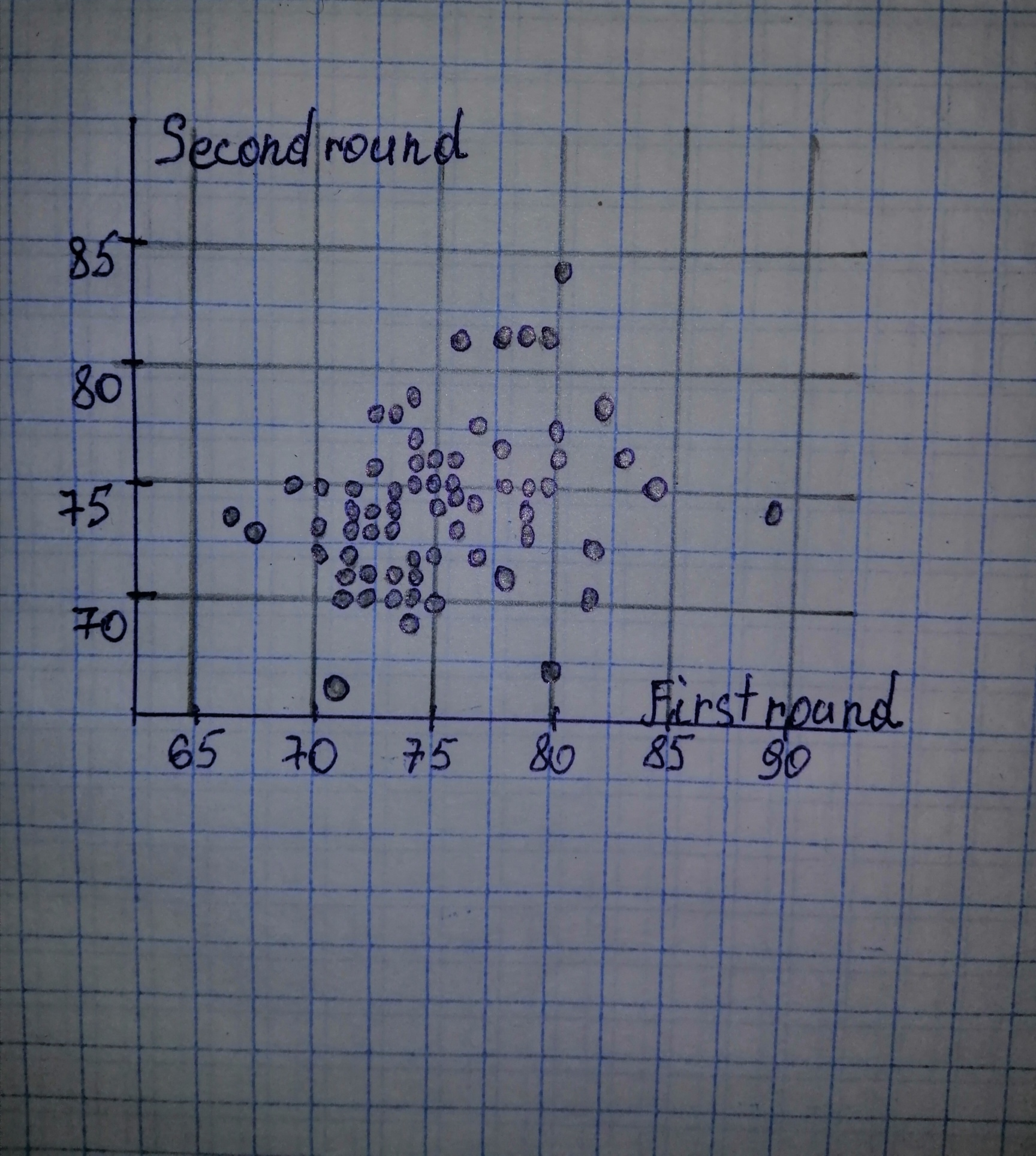# Use the technology of your choice to do the following tasks. In the article “Statistical Fallacies in Sports” (Chance, Vol. 19, No. 4, pp. 50-56), S.Armorikam 2021-02-22 Answered
Use the technology of your choice to do the following tasks. In the article “Statistical Fallacies in Sports” (Chance, Vol. 19, No. 4, pp. 50-56), S. Berry discussed, among other things, the relation between scores for the first and second rounds of the 2006 Masters golf tournament. You will find those scores on the WeissStats CD. For part (d), predict the secondround score of a golfer who got a 72 on the first round. a) Construct and interpret a scatterplot for the data. b) Decide whether finding a regression line for the data is reasonable. If so, then also do parts (c)–(f). c) Determine and interpret the regression equation. d) Make the indicated predictions. e) Compute and interpret the correlation coefficient. f) Identify potential outliers and influential observations.
You can still ask an expert for help

• Live experts 24/7
• Questions are typically answered in as fast as 30 minutes
• Personalized clear answers

Solve your problem for the price of one coffee

• Math expert for every subject
• Pay only if we can solve itestenutC

Given: a) First round is on the horizontal axis and Second round is on the vertical axis.b) When there is no strong curvature presents in the scatterplot, then it is safe to assume that there is a linear relationship between the variables and thus it is then reasonable to find a regression line. We note that the scatterplot of part (a) does not contain strong curvature and thus is reasonable to find the regression line. c) We determine all necessary sums:

Next, we can determine

The estimate b of the slope
The mean is the sum of all values divided by the number of values:
The estimate a of the intercept
General least-squares equation:

d) Let us evalute the regression line in part (c) at

We have step-by-step solutions for your answer!